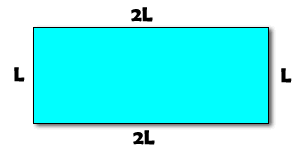# Use algebra to calculate width and length

### Question:

A piece of wire 42 cm long is bent into the shape of a rectangle whose width is twice its length. Find the dimensions of the rectangle.

There are two ways to approach this problem. First, you could solve it with a set of equations. The first equation below describes the perimeter of the rectangle. Since we are given that the piece of wire is 42 cm long, and the wire forms the outside of the rectangle, that must be the perimeter. The perimeter is 42 cm. Recall that the perimeter is calculated as 2L + 2W because there are two width sides and two length sides in any rectangle.

$$2W+2L=42$$

The second equation, below, describes the other constraint given in the problem. It says that the width is twice the length, so we can translate that into an equation. Width is twice the length:

$$W=2L$$

We have two equations with two unknowns, so we just solve a system of equations. In this case it's pretty simple because the second equation can be directly inserted (substituted) into the first, giving us:

$$2W+2L=42$$ $$2(2L)+2L=42$$ $$4L+2L=42$$ $$6L=42$$ $$L=7$$

Since the width equals twice the length, the width must be 14.

You could also solve this problem graphically by drawing a rectangle with length L and width 2L (since the width is twice the length):We know that the perimeter is 42, and adding all the sides together gives us an equation that looks like $$2L+2L+L+L=6L$$. The perimeter equals 6L:

$$6L=42$$ $$L=7$$

Just like the first method, $$W=2L$$ so $$W=14$$.